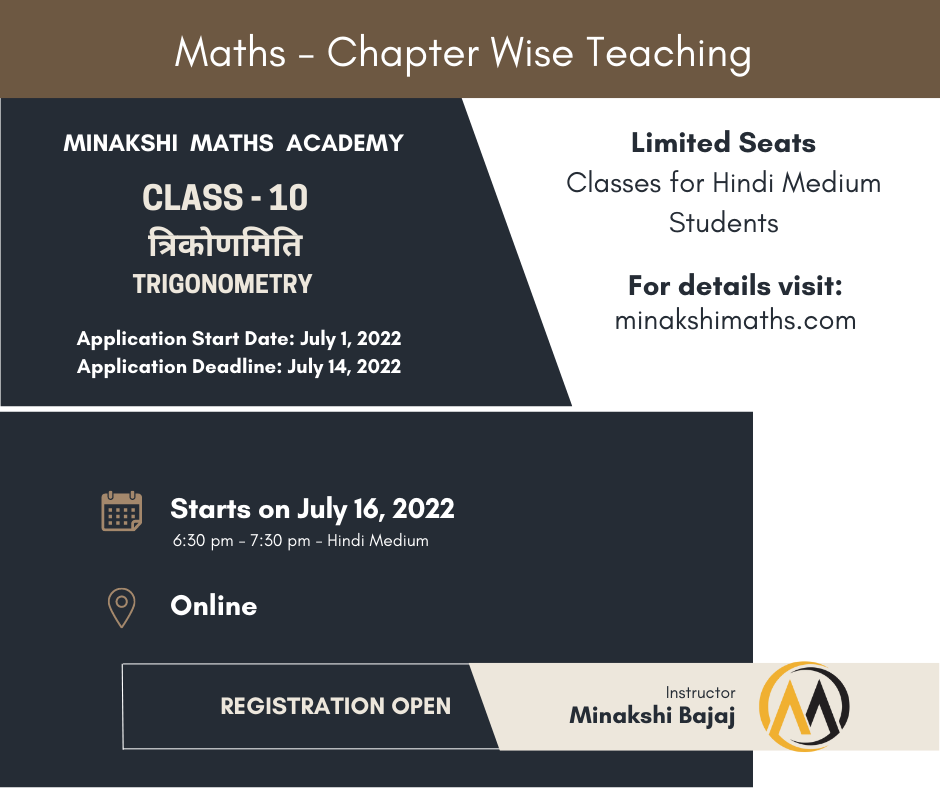## Announcement

##### Announcements
• New Batch  – Class 10 Revision

Starts on Aug 3, 2022 – Chapters covered  (1 to 5 )

• New Batch – Trigonometry Below:• On your right-hand side, we have covered previous year’s question papers and solutions. We try to update it on regular basis. Hopefully, it will help the students know the trend being followed in making these papers. Math requires practice and going through these question papers can help them do that. We wish all the students the best of luck for their future exams.

###### PISA Test

PISA Test – Programme for International Student Assessment (PISA). OECD member countries have postponed the PISA 2021 to PISA 2022 because of the COVID difficulties.

It is a program under OECD that measures 15 years of age students on reading, mathematics, and science literacy every 3 years.

OECD stands for  Organisation for Economic Cooperation and Development. It promotes policies that will improve the economic and social well-being of people around the world.

India took part in Pisa 2009 and got 72nd position among 74 participating countries. Thereafter, the government boycotted PISA. A new agreement is signed in 2019 between India and OECD as per which India will participate in PISA 2022. Since questions are as per international benchmarks, the idea is to improve the quality of education in India and to bring about competent examination reforms.

https://www.oecd.org/pisa/

For Mathematics questions asked in PISA 2012, 2006, and 2003, click on the link below:

https://www.oecd.org/pisa/pisaproducts/pisa-test-questions.htm

###### Joint Entrance Examination – MCQs with SOLUTIONS

To score high marks, students need to understand the pattern and type of questions that are normally asked ( JEE Mains MCQs ). We have provided here chapter-wise MCQs along with their SOLUTIONS that were asked in previous years. It is advised that students must go through these for the examination.

Recently there have been some important changes. From now onwards the exam will not be held in pen-paper mode. It will be conducted online. But here we have covered all the questions that were asked in previous years along with SOLUTIONS – in both modes i.e pen-paper mode (offline mode) and online mode. Students can also DOWNLOAD Questions with SOLUTIONS from here.

2. Trigonometry – HERE

3. Inverse Trigonometry – HERE

4. Binomial – HERE

5. Differential Equations – HERE

6. Three Dimensional Geometry – HERE

7. Limits and Continuity – HERE

8. Indefinite Integration – HERE

9. Progressions – HERE

10. Matrices and Determinants – HERE

11. Complex Numbers – HERE

12. Application of Integration – HERE

13. Circle – HERE

14. Straight Lines – HERE

15. Probability – HERE

16. Permutation and Combination – HERE

17. Application of Derivatives – HERE

18. Sets, Relations, and Functions – HERE

19. Definite Integrals – HERE

20. Vectors – HERE

###### Previous Year Questions Papers with Solutions – Class 10

1. Download maths question paper (BASIC) for class 10,  2020 (CBSE) – Set 1 FROM HERE

2. Download maths question paper (BASIC) for class 10, 2020 (CBSE) – Set 2 from HERE

3. Download maths question paper (BASIC) for class 10, 2020 (CBSE) – set 3 from HERE

4. Download maths question paper (STANDARD) for class 10, 2020 – (CBSE) set 1 FROM HERE

5. Download maths question paper (STANDARD) for class 10, 2020 (CBSE) – set 2 from HERE

6. Download maths question paper (STANDARD) for class 10, 2020 (CBSE) – set 3 from HERE

7.  CBSE class 10 maths solutions 2020 (standard) – set 1 HERE

8.  CBSE class 10 maths solutions 2020 (standard) – set 2 HERE

9.  CBSE class 10 maths solutions 2020 (standard) – set  3 HERE

10.  CBSE class 10 maths solutions 2020 (BASIC) – set 1 HERE

11.   CBSE class 10 maths solutions 2020 (BASIC) – set 2 HERE

12.   CBSE class 10 maths solutions 2020 (BASIC) – set 3 HERE

CBSE JULY 2019 COMPARTMENT

1.  Download MATHS question paper (COMPARTMENT) for class 10, 2019 (CBSE) – set 1 FROM HERE

2.  Download MATHS question paper (COMPARTMENT) for class 10, 2019 (CBSE) – set 2 from HERE

3.  Download MATHS question paper (COMPARTMENT) for class 10,  2019 (CBSE) – set 3 from HERE

CBSE 2019

1.  Download MATHS question paper for class 10, 2019 (CBSE) – set 1 HERE

2.  Download MATHS question paper for class 10, 2019 (CBSE) – set 2 HERE

3.  Download MATHS question paper for class 10, 2019 (CBSE) – set 3 HERE

4.  CBSE class 10 maths solutions 2019 – set 1 HERE

5.  CBSE class 10 maths solutions 2019 – set 2 HERE

###### Previous Year Questions Papers with Solutions – Class 12

1.  Download Maths question paper – class 12, 2020 (CBSE) Set 3 –  from HERE

2.  Download Maths Solutions – class 12, 2020 (CBSE) Set 3 from HERE

3.  Download  Maths question paper –  class 12, 2019 (CBSE) Set 1 –  from HERE

4.  Download Maths question paper – class 12, 2019 (CBSE) Set 3 –  from HERE

5.  Download  Maths Solutions – class 12, 2019 (CBSE) Set 1- from HERE

6.  Download  Maths Solutions – class 12, 2019 (CBSE) Set 3 –  from HERE

###### Keep Yourself Updated with News###### Click the above pic – Not to Miss
Select the fields to be shown. Others will be hidden. Drag and drop to rearrange the order.
• Image
• SKU
• Rating
• Price
• Stock
• Availability